# Solution assignment 04 Tangent line to graph

### Assignment 4

Calculate the equation of the line through the point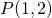with slope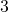.

### Solution

We can calculate the equation of this line by using the following formula: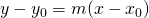with: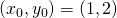and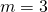and thus we get: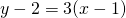or: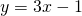which is the equation of the required line.